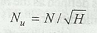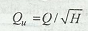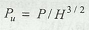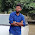### Unit Speed, Unit Discharge and Unit Power

What is Unit Speed, Unit Discharge and Unit Power of a turbine? How do we calculate these using formula?

The unit speed is the speed of the turbine operating under one meter head. Mathematically, unit speed,The unit discharge is the discharge through a turbine when the head on the turbine is unity. Mathematically, unit discharge,The unit power is the power developed by a turbine when the head on the turbine is unity. Mathematically, unit power,1.2.3.